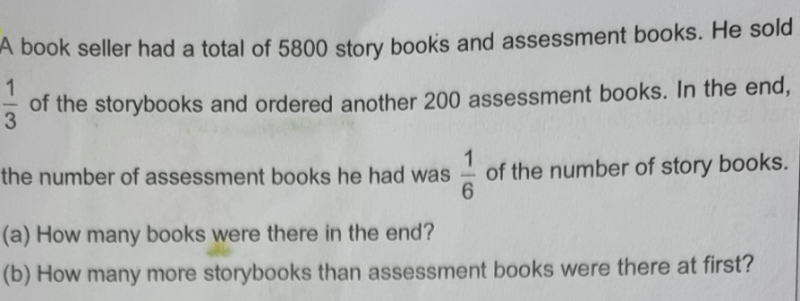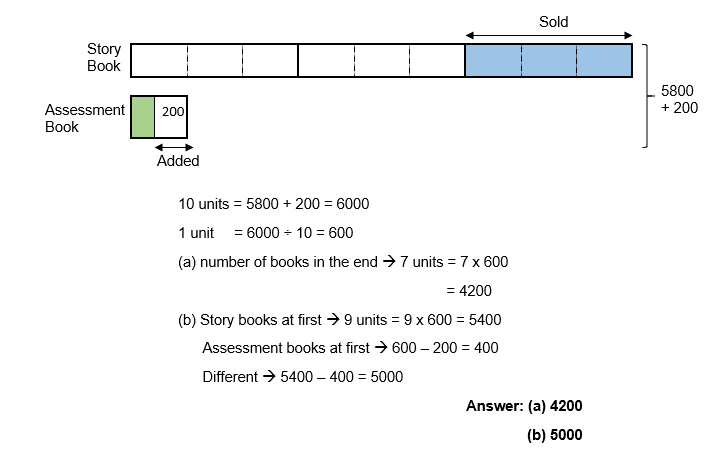# QuestionIs this a changed total type of question? Any help to solve it will be appreciated. Tks!

In the end, ratio of assessment books to story books is 1 : 6

Working backwards to find initial number of books:

Subtract the 200 assessment books bought and add back the 1/3 of story books sold (6 units is 2/3, initial would be 9 units). The initial ratio would then be 1u – 200 : 9 u.

Equate the initial total number of books to be 5800:

10 u – 200 = 5800

10 u = 6000

u = 600.

(a) Total number of books at the end = 7 u

= 7 x 600

= 4200

(b) Initial number of assessment books = 1 u – 200

= 600 – 200

= 400

Initial number of story books = 9 u

= 9 x 600

= 5400

Difference = 5400 – 400

= 5000 //

0 Replies 0 Likes